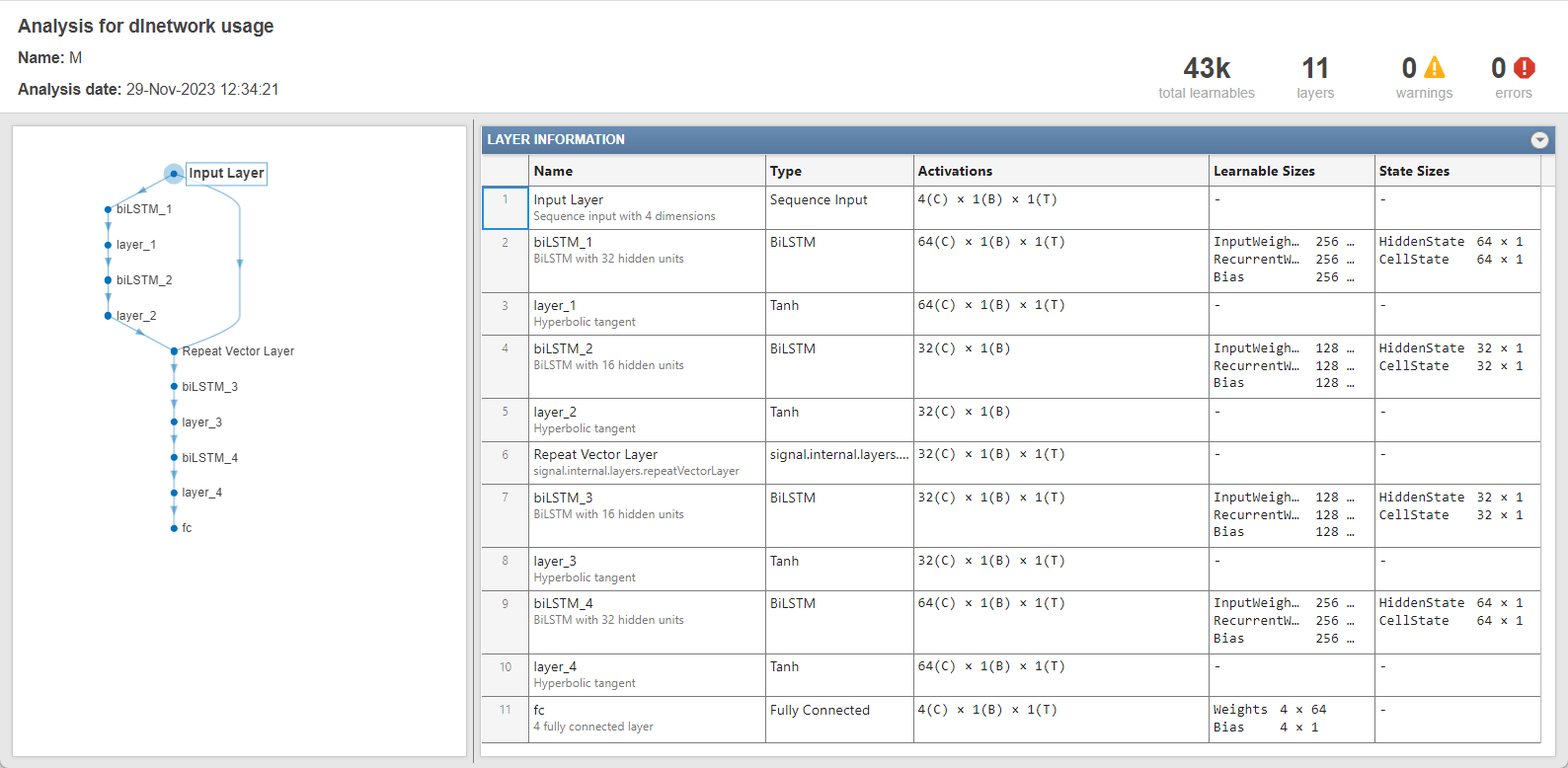# getModel

Get underlying neural network model of signal anomaly detector

Since R2023a

## Syntax

``m = getModel(d)``

## Description

example

````m = getModel(d)` returns the underlying model of the signal anomaly detector `d`. `m` is a `dlnetwork` and initialized when the detector is trained.```

## Examples

collapse all

Create an object that uses a long short-term memory (LSTM) autoencoder model to detect anomalies in four-channel signals. Use `deepSignalAnomalyDetector` with default property values.

`D = deepSignalAnomalyDetector(4,"lstm");`

Get the underlying neural network model of the LSTM anomaly detector. Use the `analyzeNetwork` (Deep Learning Toolbox) function to inspect the model.

```M = getModel(D); analyzeNetwork(M)```## Input Arguments

collapse all

Anomaly detector, specified as a `deepSignalAnomalyDetectorCNN` or `deepSignalAnomalyDetectorLSTM` object. Use the `deepSignalAnomalyDetector` function to create `d`.

## Output Arguments

collapse all

Underlying neural network model of detector `d`, returned as a `dlnetwork` (Deep Learning Toolbox) object.

## Version History

Introduced in R2023a